## 4.5.1 Semi-Infinite CNT Contacts

Figure 4.8 shows carbon rings of A or B-type coupled to a semi-infinite CNT acting as a contact. Each circle (rectangle) represents a carbon ring consisting of A or B-type carbon atoms. The carbon ring couples to the nearest ring, with a coupling matrix of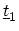or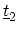, and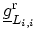is the surface GREEN's function for the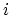th ring in the left extension, ordered from the channel-contact interface. The recursive relation (G.22) can be applied to the CNT in Fig. 4.8 and gives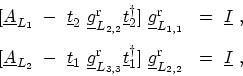(4.31)

where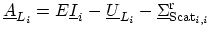(see Appendix G.1), andandare given by (4.17) and (4.18), respectively. Since the potential is invariant inside the contact,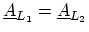. Furthermore,due to the periodicity of the CNT lattice. Using these relations, (4.31) represent two coupled matrix equations with two unknowns,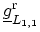and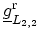, which can be solved by iteration. However, in mode-space representation matricesandare replaced by the numbersand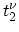, respectively. As a result, the surface GREEN's function for each mode can be calculated analytically by solving a quadratic equation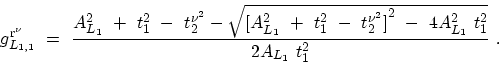(4.32)

The self-energy of the left contact for the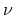th mode is therefore given by(4.33)

A similar relation holds for the right contact self-energy.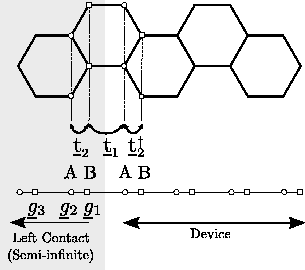M. Pourfath: Numerical Study of Quantum Transport in Carbon Nanotube-Based Transistors